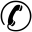All India Help Line - 09999265274,09999736252

nbsp;nbsp;
nbsp;
nbsp;

# How does IBPS calculate TWSS

TWSS scores are calculated by linear transformation method taking mean score = 25 and standard deviation = 5. Still many candidates unable to understand this formula. Find below TWSS explanation with an example.

1) Actual corrected score: Number of questions you attempted correctly minus negative marking (0.25 marks for question). For example Ram attempted 25 questions in reasoning section out of which 4 were wrong. His corrected score will be 21- 1 = 20.

2) Equated score: Scores of candidates of every slot and paper set is equated according to difficulty level of the test. Difficulty level of the test is decided by marks scored by all the candidates in a particular question paper.
For example there 50 question paper set in IBPS PO 2013 (Just an example to explain the concept). In set 21 average marks of students is lower than average marks of students in other question sets. Automated computer program will equate the scores of all the candidates of set 21 by multiplying it with a equating value, for example 1.0122 or 0.986

3) Final standardized score: As announced by IBPS in their official website that they use Linear transformation method by taking mean = 25 and standard deviation = 5, to calculate TWSS of candidates.